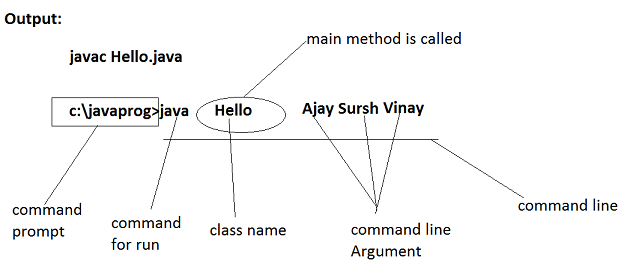# Java Programing laungage

Command Line Argument in Java
 Previous Home Next

Sometimes we will want to pass information into a program when we run it. The arguments passed from the console can be received in the java program and it can be used as an input. This is accomplished by passing command-line argument to main(). A command line argument is the information that directly follows the program's name n the command line which it is executed. To access the command-line argument inside a java program we have to use string array passed to main().

The JVM calss the main() method and passes the command line argument to it as an array of string. The length of the array(i.e the number of the command line arguments) is obtained using attribute length.

All java applications contain a static method named main(). It takes one argument that is an array of String objects. These objects represent any arguments that may have been entered by the user on the command line. The number of command line arguments is obtained by the expression args.length. It is of type int. We can access individual arguments by args, args and so on...

### Example :

```class Hello
{
public static void main(String args[])
{
for(int i=0;i<args.length;i++)
{
System.out.println("Hello"+args[i]);
}
}
}```

### output :Converting String to int

A Numeric string can be converted into an integer using the parseInt method in the Integer class.The parseInt method is invoked as follows:

`Integer.parseInt( NumericString );`

The parseInt method returns an integer value of the numeric string.

### Example :

```class Sum
{
public static void main(String args[])
{
int sum=0;
for(int i=0;i<args.length;i++)
{
sum+=Integer.parseInt(args[i]);

}
System.out.printf("sum is %d",sum);
}
}```
 Previous Home Next